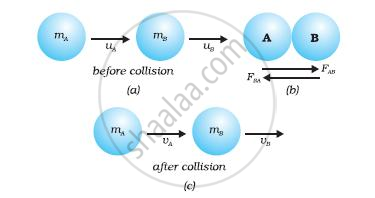# Conservation of Momentum

#### notes

Law of conservation of Momentum:
From Newtons third law of motion we know that whenever a force is applied on a body there will be an equal and opposite reaction. Action and reaction forces result in change in velocities of both the bodies which in turn change the momentum of the bodies.

In an elastic collision the initial momentum of the bodies before collision is found to be equal to the final momentum of the bodies after collision.

Law of conservation of motion states that if a group of bodies are exerting force on each other. ie. Interacting with each other, their total momentum remains constant before and after the collision provided there is no external force acting on them.Suppose two objects (two balls A and B, say) of masses mA and mB are travelling in the same direction along a straight line at different velocities uA and uB, respectively [Fig.(a)]. And there are no other external unbalanced forces acting on them. Let uA > uB and the two balls collide with each other as shown in Fig.(b). During collision which lasts for a time t, the ball A exerts a force FAB on ball B and the ball B exerts a force FBA on ball A. Suppose vA and vB are the velocities of the two balls A and B after the collision, respectively [Fig.(c)].

the momenta (plural of momentum) of ball A before and after the collision are mAuA and mAvA, respectively. The rate of change of its momentum (or FAB) during collision will be

"m"_"A"("v"_"A" - "u"_"A")/"t"

Similarly, the rate of change of momentum of ball B=(FBA) during the collison will be

"m"_"B"("v"_"B" - "u"_"B")/"t"

According to the third law of motion, the force FAB exerted by ball A on ball B and the force FBA exerted by the ball B on ball A must be equal and opposite to each other. Therefore, FAB = – FBA or

"m"_"A"("v"_"A" - "u"_"A")/"t"= "m"_"B"("v"_"B" - "u"_"B")/"t"

This gives,
mAuA + mBuB = mAvA + mBvB

Since (mAuA + mBuB) is the total momentum of the two balls A and B before the collision and (mAvA + mBvB) is their total momentum after the collision, we observe that the total momentum of the two balls remains unchanged or conserved provided no other external force acts. As a result of this ideal collision experiment, we say that the sum of momenta of the two objects before collision is equal to the sum of momenta after the collision provided there is no external unbalanced force acting on them. This is known as the law of conservation of momentum. This statement can alternatively be given as the total momentum of the two objects is unchanged or conserved by the collision.

If you would like to contribute notes or other learning material, please submit them using the button below.

### Shaalaa.com

Conservation of momentum [00:15:34]
S
0%